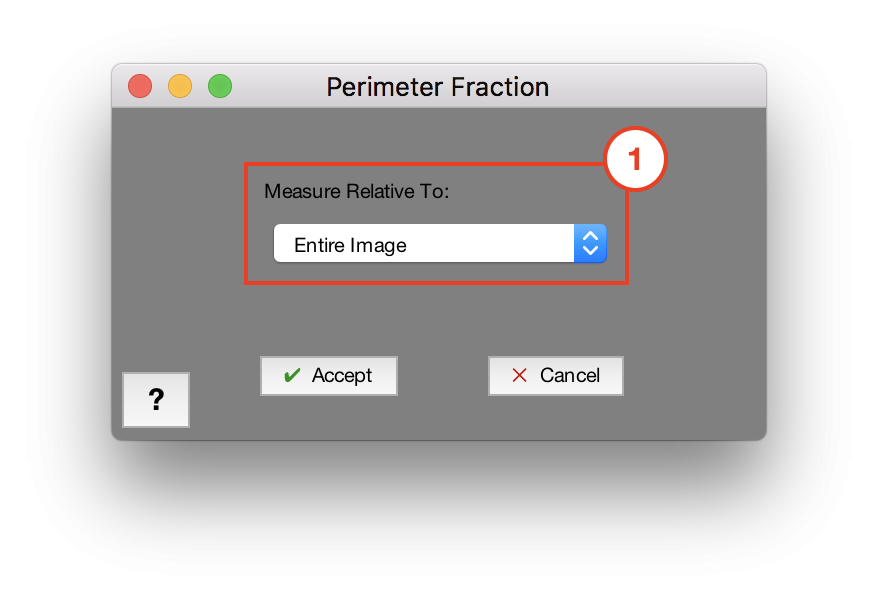### Measurements > Perimeter Fraction

Measures the total perimeter fraction of features in the current image. This is calculated as the total perimeter length divided by the either the total number of pixels in the image, or the total number of selected pixels in a chosen Memory Image.### 1. Measure Relative To

Choose to measure relative to either the entire image, or the selection in a Memory Image

Need more help with this?
Chat with an expert now ››